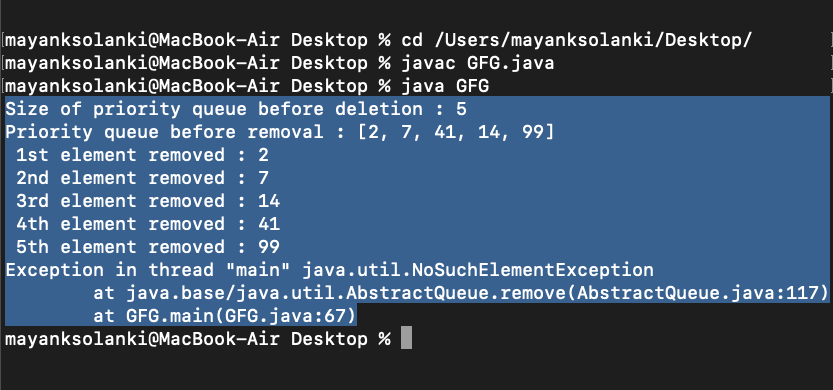# PriorityQueue remove() Method in Java

• Difficulty Level : Medium
• Last Updated : 14 Dec, 2021

The remove() method of PriorityQueue class of java.util package is used to remove a particular element from a PriorityQueue. As we all know that the elements while entering into the priority queue are not sorted but as we all know while taking out elements from the priority queue the elements are always sorted being a trait of the priority queue. Here the default ordering of priority of elements for data types is defined as follows:

• Integer: Smallest elements that come first (while dealing with positive numbers only)
• String: Alphabetical ordering

Note: We can also insert a Comparator while creating an instance of this class which tells us how the priority should be defined.

Syntax:

`PriorityQueue<String> = new PriorityQueue<String>(ComparatorHere);`

Syntax: Remove method

`Priority_Queue.remove(Object O)`

Parameters: The parameter O is of the type of PriorityQueue and specifies the element to be removed from the PriorityQueue.

Return Value: This method returns True if the specified element is present in the Queue else it returns False.

Example 1

## Java

 `// Java Program to Illustrate remove() Method` `// in PriorityQueue` `// Where Elements are of String Type`   `// Importing all utility classes` `import` `java.util.*;`   `// Main class` `// PriorityQueueDemo` `public` `class` `GFG {`   `    ``// Main driver method` `    ``public` `static` `void` `main(String args[])` `    ``{`   `        ``// Creating an empty PriorityQueue` `        ``// where elements are of string type` `        ``PriorityQueue queue` `            ``= ``new` `PriorityQueue();`   `        ``// Adding elements into the Queue` `        ``// using add() method` `        ``queue.add(``"Welcome"``);` `        ``queue.add(``"To"``);` `        ``queue.add(``"Geeks"``);` `        ``queue.add(``"For"``);` `        ``queue.add(``"Geeks"``);`   `        ``// Printing the elements of PriorityQueue` `        ``System.out.println(``"Initial PriorityQueue: "` `                           ``+ queue);`   `        ``// Removing elements from PriorityQueue` `        ``// using remove() method` `        ``queue.remove(``"Geeks"``);` `        ``queue.remove(``"For"``);` `        ``queue.remove(``"Welcome"``);`   `        ``// Displaying the PriorityQueue` `        ``// after removal of element` `        ``System.out.println(``"PriorityQueue after removing "` `                           ``+ ``"elements: "` `+ queue);` `    ``}` `}`

Output

```Initial PriorityQueue: [For, Geeks, To, Welcome, Geeks]
PriorityQueue after removing elements: [Geeks, To]```

Example 2

## Java

 `// Java Program to Illustrate remove() Method` `// of PriorityQueue class` `// Where Elements are of Integer type`   `// Importing required classes` `import` `java.util.*;`   `// Main class` `// PriorityQueueDemo` `public` `class` `GFG {`   `    ``// Main driver method` `    ``public` `static` `void` `main(String args[])` `    ``{`   `        ``// Creating an empty PriorityQueue by` `        ``// creating an object of integer type` `        ``PriorityQueue queue` `            ``= ``new` `PriorityQueue();`   `        ``// Adding custom input elements` `        ``// using add() method` `        ``queue.add(``10``);` `        ``queue.add(``15``);` `        ``queue.add(``30``);` `        ``queue.add(``20``);` `        ``queue.add(``5``);`   `        ``// Displaying the PriorityQueue` `        ``System.out.println(``"Initial PriorityQueue: "` `                           ``+ queue);`   `        ``// Removing elements from the PriorityQueue` `        ``// using remove() method` `        ``queue.remove(``30``);` `        ``queue.remove(``5``);`   `        ``// Displaying the PriorityQueue elements` `        ``// after removal` `        ``System.out.println(``"PriorityQueue after removing "` `                           ``+ ``"elements: "` `+ queue);` `    ``}` `}`

Output:

```Initial PriorityQueue: [5, 10, 30, 20, 15]
PriorityQueue after removing elements: [10, 20, 15]```

Geek, have you ever wondered what will happen if calls of remove() method exceed the elements present in the queue. In this scenario, it will continue to remove the elements that were there, and thereafter it will not find any element to remove priority-wise, so it will throw an exception which is as follows.

Note: This class do implements AbstractQueueInterface

Example

## Java

 `// Java Program to illustrate remove() Method` `// in PriorityQueue` `// Where Exception is encountered`   `// Importing required classes` `import` `java.io.*;` `import` `java.util.PriorityQueue;`   `// Main class` `// PriorityQueueException` `class` `GFG {`   `    ``// Main driver method` `    ``public` `static` `void` `main(String[] args)` `    ``{`   `        ``// Creating an empty PriorityQueue` `        ``PriorityQueue pq` `            ``= ``new` `PriorityQueue();`   `        ``// Note: Elements are inserted in unsorted order in` `        ``// priority queue but after removal of elements` `        ``// queue is always sorted.`   `        ``// Adding elements in above queue` `        ``// using add() method` `        ``pq.add(``2``);` `        ``pq.add(``14``);` `        ``pq.add(``41``);` `        ``pq.add(``7``);` `        ``pq.add(``99``);`   `        ``// Elements in queue are unsorted by far`   `        ``// Getting size of above queue before deletion` `        ``// of any element using size() method` `        ``System.out.println(` `            ``"Size of priority queue before deletion : "` `            ``+ pq.size());`   `        ``// Printing all elements of above queue` `        ``System.out.println(` `            ``"Priority queue before removal : "` `+ pq);`   `        ``// Calling remove() method over priority queue` `        ``// in which there were 5 elements`   `        ``// Here calling remove() method say be it 2 times` `        ``// So 2 top priority elements will be removed` `        ``System.out.println(``" 1st element removed : "` `                           ``+ pq.remove());` `        ``System.out.println(``" 2nd element removed : "` `                           ``+ pq.remove());` `        ``System.out.println(``" 3rd element removed : "` `                           ``+ pq.remove());` `        ``System.out.println(``" 4th element removed : "` `                           ``+ pq.remove());` `        ``System.out.println(``" 5th element removed : "` `                           ``+ pq.remove());`   `        ``// By now queue is empty and if now we made further` `        ``// remove() call it will throw exception for this` `        ``System.out.println(``" 6th element removed : "` `                           ``+ pq.remove());`   `        ``// As we know smaller the integer bigger the` `        ``// priority been set by default comparator of this` `        ``// class`   `        ``// Note: Now the element is always returned sorted` `        ``// from a priority queue is a trait of this class`   `        ``// Printing the queue after removal of priority` `        ``// elements` `        ``System.out.println(` `            ``"Priority queue after removal as follows: "` `            ``+ pq);` `    ``}` `}`

Output:Output explanation:

It is showcasing that there are no further elements left in the queue as a queue is empty by now so does it throw NoSuchElementException.

My Personal Notes arrow_drop_up
Recommended Articles
Page :